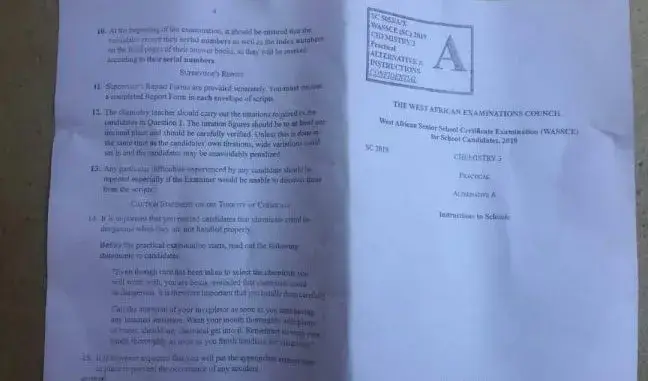# 2023 WASSCE: Likely Elective Chemistry Questions## Solve these 2023 WASSCE Chemistry questions which are likely to drop in the forthcoming WASSCE. These 2023 WASSCE Chemistry questions are well-analyzed.

Candidates are advised to solve all the questions and seek help when they find difficulty.

PAPER 2

1. a. Describe a test to distinguish between C2H2 and C2H4.

b. State two factors that influence the preferential discharge of ions during electrolysis.

c. Consider the following ionic equations:

MnO4- (aqueous) = Mn2+

Write the complete balanced ionic equation

d. State two(2) reasons why aluminum is used in packaging foods

e. Define the term ‘solubility of a salt’

f. Using the Kinetic theory, explain what happens when a solid is heated.

g. What is a ‘substitution reaction’?

h. Calculate the percentage by mass of oxygen in magnesium trioxonitrate(V)

Take (N=14.0, O= 16.0, Mg= 24.0)

i. Name two indicators used in quantitative analysis.

f. State two differences between ionization energy and electron affinity.

g. State:

i. Charles Law ii. Periodic Law iii. Boyles Law

2. a. i. Write a complete chemical balanced equation for the preparation of hydrogen chloride using concentrated H2SO4.

ii. State two advantages of hard water.

b. i. In the extraction process of aluminum, explain briefly how bauxite is first converted to pure alumina.

ii. State four (4) properties of metals which can be accounted for by their structure.

c. Describe the industrial preparation of oxygen

d. State two uses of oxygen

3. Gasification of coke involves the reaction of coke with air and steam at high temperatures:

i. Explain the process that occurs when coke reacts with air

ii. Name the resulting product

iii. Write a complete balanced chemical equation for the reaction of coke with steam.

iv. State two uses of the product named in 3.ii

v. State two advantages of the product named in 3.ii

4. List three(3) important properties of calcium

a. State two(2) uses of calcium.

These are well analyzed questions. Taken from the predicted topics and are likely to drop in the 2023 WASSCE. We wish all 2023 WASSCE candidates the very best of luck.

READ ALSO: 2023 BECE Candidates Looking for Mock Exam Apor: Proofs